﻿ 基于小波包振动谱图的柴油机在线故障诊断方法
 舰船科学技术2016, Vol. 38Issue (8): 128-133PDF

Diesel engine online fault diagnosis method based on wavelet packet vibration image
YUE Ying-juan, SUN Gang, CAI Yan-ping
The Rocket Force Engineering University, Xi'an 710025 China
Abstract: Diesel engine is the main power device of ship, for the disadvantages of traditional methods can't effectively extract fault feature and can't realize the fault online diagnosis, this paper proposes a new diesel engine online fault diagnosis method based on wavelet packet vibration image. Firstly, using the wavelet packet to the collected diesel engine vibration acceleration signals to get vibration images; then using bilinear interpolation method in the generated vibration images for data dimension reduction, then using the gray level co-occurrence matrix for the texture feature parameter extraction; finally with classifier accomplish fault diagnosis. This method was applied to fault diagnosis of diesel engine valve clearance, the results show that the fault can be quickly and efficiently diagnosed by diesel engine online fault diagnosis method based on wavelet packet vibration image, recognition accuracy rate can reach 99.17 percent, only consuming 0.24 S, explored a new way for diesel engine online fault diagnosis.
Key words: diesel engine     wavelet packet     vibration image     bilinear interpolation method     gray level co-occurrence matrix
0 引言

1 小波包振动谱图像的生成

 ${{y}_{2n}}(t)=\sqrt{2}\sum{h(k){{y}_{n}}\left( 2t-k \right)},$ (1)
 ${{y}_{2n+1}}(t)=\sqrt{2}\sum{g(k){{y}_{n}}\left( 2t-k \right)}\circ$ (2)

2 振动谱图像的特征参数提取

2.1 图像压缩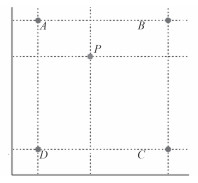图 1 双线性内插值简图 Fig. 1 Schematic Diagram of Quadratic Interpolation Method

4）熵、和熵及差熵。代表图像的信息量，是图像内容随机性的量度，指示纹理的复杂程度。当图像复杂程度高时，此时熵值最大，分形值也相对较高；当图像复杂程度低时，熵值较小或为 0。

 ${{f}_{\text{4}}}=-\sum\limits_{k=2}^{2{{N}_{g}}}{{{P}_{x+y}}(k)\log \left[ {{P}_{x+y}}(k) \right]};$ (11)

 ${{f}_{\text{5}}}=-\sum\limits_{i=1}^{{{N}_{g}}}{\sum\limits_{j=1}^{{{N}_{g}}}{{{P}^{2}}(i,j,d,\theta )}}\log \left[ P(i,j,d,\theta ) \right];$ (12)

 ${{f}_{\text{6}}}=-\sum\limits_{k=0}^{{{N}_{g}}-1}{{{P}_{x-y}}(k)\log \left[ {{P}_{x-y}}(k) \right]}\circ$ (13)

5）均值和。图像区域内像素点平均灰度值的度量，反映图像整体色调的明暗深浅。

 ${{f}_{\text{7}}}=\sum\limits_{k=2}^{2{{N}_{g}}}{k\times {{P}_{x+y}}(k)}\circ$ (14)

6）方差、方差和。反映纹理变化快慢、周期性大小的物理量。值越大，表明纹理周期越大。方差、方差和的值均随图像纹理的不同有较大的变异，可作为区分纹理的一个重要指标。

 ${{f}_{\text{8}}}=\sum\limits_{i=1}^{{{N}_{g}}}{\sum\limits_{j=1}^{{{N}_{g}}}{{{(1-u)}^{2}}P(i,j,d,\theta )}},$ (15)

 ${{f}_{\text{9}}}=\sum\limits_{k=2}^{2{{N}_{g}}}{{{(k-{{f}_{\text{7}}})}^{2}}{{P}_{x+y}}(k)}\circ$ (16)

7）差的方差。表明邻近像素对灰度值差异的方差，对比越强烈，值越大；反之，值越小。

 ${{f}_{\text{10}}}=\sum\limits_{k=0}^{{{N}_{g}}-1}{\left[ k-\sum\limits_{k=1}^{{{N}_{g}}-1}{k\times {{P}_{x-y}}(k)} \right]}\times {{P}_{x-y}}(k)\circ$ (17)

8）逆差矩。反映纹理的规则程度。纹理杂乱无章、难于描述的，逆差矩值较小；规律较强、易于描述的，逆差矩值较大。

 ${{f}_{\text{11}}}=\sum\limits_{i=1}^{{{N}_{g}}}{\sum\limits_{j=1}^{{{N}_{g}}}{P(i,j,d,\theta )}}/\left[ 1+{{(i-j)}^{2}} \right]\circ$ (18)

 $t=[{{f}_{1}},{{f}_{2}},{{f}_{3}},{{f}_{4}},{{f}_{5}},{{f}_{6}},{{f}_{7}},{{f}_{8}},{{f}_{9}},{{f}_{10}},{{f}_{11}}]\circ$ (19)
3 基于振动谱图像的故障诊断流程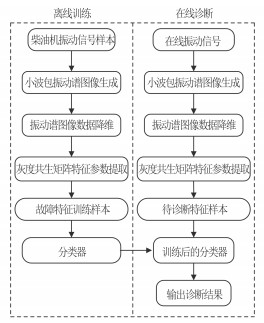图 2 基于小波包振动谱图的柴油机在线故障诊断步骤 Fig. 2 Diesel Engine Online Fault Diagnosis Method Based on Wavelet Packet Vibration Image
4 柴油机故障诊断实例 4.1 柴油机实验工况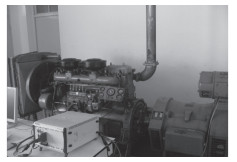图 3 试验平台 Fig. 3 Experimental Platform表 1 四种实验工况设置（单位：mm） Tab.1 Four states of IC engine’s valve train (mm)
4.2 小波包振动谱图像生成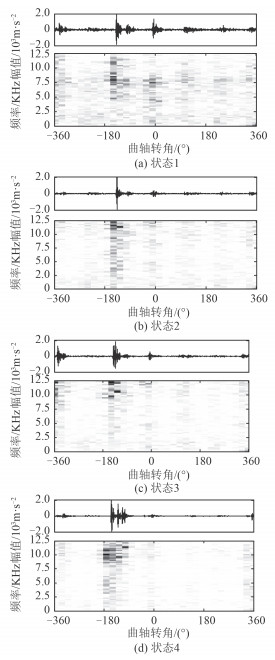图 4 四种典型工况下的小波包振动谱图像 Fig. 4 Wavelet Packet Vibration Image for Four States

4.3 振动谱图像数据降维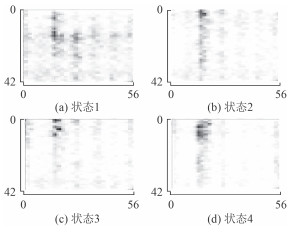图 5 数据降维后的小波包振动谱图像 Fig. 5 Wavelet Packet Vibration Images after Data Dimension Reduction
4.4 灰度共生矩阵特征提取表 2 降维和灰度级对振动谱图像识别耗时的影响（单位：s） Tab.2 Feature Parameters Extracted By Gray Level Co-Occurrence Matrix
4.5 柴油机故障分类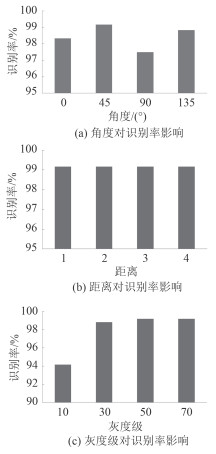图 6 灰度共生矩阵不同特征参数对识别率影响 Fig. 6 The Influence of Gray Level Co-Occurrence Matrix Parameters to Recognition Rate表 3 采用灰度共生矩阵提取的特征参量 Tab.3 The Influence of Dimensionality Reduction and Gray Level to The Time Consuming (s)
5 结语

1）小波包振动谱图能够有效地反映柴油机气门间隙不同工况下时频分布特点，可用于对柴油机气门间隙故障的判别。

2）用双线性内插值算法对生成的振动谱图进行压缩，能够在极大较小计算量的同时保留图像的敏感信息。

3）用灰度共生矩阵对得到的振动谱图进行特征参数提取，并用分类器进行分类，完成故障诊断。将该方法应用于柴油机气门间隙的故障诊断中，结果表明，文中提出的基于小波包振动谱图像的柴油机在线故障诊断方法识别精度高，耗时短，适用于柴油机气门间隙的故障诊断，可实现舰船柴油机的在线监测诊断。

  DURAK L, ARIKAN O. Short-time Fourier transform: Two fundamental properties and an optimal implementation[J]. IEEE Transactions on Signal Processing , 2003, 51 (5) :1231–1242. DOI:10.1109/TSP.2003.810293  DJUROVIĆ I, SEJDIĆ E, JIANG J. Frequency-based window width optimization for S-transform[J]. AEU-international Journal of Electronics and Communications , 2008, 62 (4) :245–250. DOI:10.1016/j.aeue.2007.03.014  SMITH C, AKUJUOBI C M, HAMORY P, et al. An approach to vibration analysis using wavelets in an application of aircraft health monitoring[J]. Mechanical Systems and Signal Processing , 2007, 21 (3) :1255–1272. DOI:10.1016/j.ymssp.2006.06.008  QAZI S, GEORGAKIS A, STERGIOULAS L K, et al. Interference suppression in the Wigner distribution using fractional Fourier transformation and signal synthesis[J]. IEEE Transactions on Signal Processing , 2007, 55 (6) :3150–3154. DOI:10.1109/TSP.2007.893971  MARGENAU H, HILL R N. Correlation between measurements in quantum theory[J]. Progress of Theoretical Physics , 1961, 26 (5) :722–738. DOI:10.1143/PTP.26.722  王珍, 马孝江. 局域波时频法在柴油机缸套活塞磨损诊断中的应用研究[J]. 内燃机学报 , 2002, 20 (2) :157–160.  蔡艳平, 李艾华, 王涛, 等. 基于 EMD-Wigner-Ville 的内燃机振动时频分析[J]. 振动工程学报 , 2010, 23 (4) :430–437.  孙斌, 万鹏威, 陶达, 等. 基于自适应最优核时频分布的鸟类识别[J]. 数据采集与处理 , 2015, 30 (6) :1187–1195.  葛哲学, 陈仲生. Matlab 时频分析技术及其应用[M]. 北京: 人民邮电出版社, 2006 .  郭亚. 振动信号处理中的小波基选择研究[D]. 合肥: 合肥工业大学, 2003.  郑红, 李钊, 李俊. 灰度共生矩阵的快速实现和优化方法研究[J]. 仪器仪表学报 , 2012, 33 (11) :2509–2515. ZHENG Hong, LI Zhao, LI Jun. Study on fast implementation and optimal methodof gray level co-occurrence matrix[J]. Chinese Journal of Scientific Instrument , 2012, 33 (11) :2509–2515.